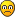## 007 Cylindrical Cross Sums SSSudoku - Walkthrough

For fans of Killer Sudoku, Samurai Sudoku and other variants

### 007 Cylindrical Cross Sums SSSudoku - Walkthrough

Hi all,

I have seen a lot of nice puzzles from quite a few people here, so I like to share a creation of myself with you in return...

I have made a very nice cylindrical sudoku, using the letter S all the time.

Since I have no webpage I can not attach the puzzle, so I give you the link to another forum where you can find it:

http://www.sudoku.org.uk/discus/messages/1289/2096.html?1153027606

Enjoy it!

Richard
Last edited by Richard S on Sun Sep 10, 2006 2:42 pm, edited 1 time in total.
Richard S

Posts: 24
Joined: 08 June 2006

### Re: 007 Cylindrical Cross Sums SSSudoku

Richard S wrote:Since I have no webpage I can not attach the puzzle, so I give you the link to another forum where you can find it ...

You could attach it by using the "url" tag with the other site's image-location:
http://www.sudoku.org.uk/discus/messages/1289/2097.jpg

Alternatively, you could use that location with the "img" tag to display it here:
[ img ]http://www.sudoku.org.uk/discus/messages/1289/2097.jpg[/img]

EDIT: Spaces added to img tag to prevent displaying it here, due to the large file size.
See udosuk's reduced-size image posted below.
Last edited by r.e.s. on Thu Jul 20, 2006 3:43 am, edited 1 time in total.
r.e.s.

Posts: 337
Joined: 31 August 2005

Thank's Udosuk,

I am not so good with computers as with puzzles...

Now that the image is on this forum, I can write the instructions here as well:

I have made a very nice cylindrical sudoku, using the letter S all the time.

Fill each column, row and outlined coloured region with the digits 0,0, 1 to 7. (The zero appears twice, to make this one more difficult.)
The numbers outside the grid are the sums of the digits you come across first: the first number (in brackets) is the number of digits you have to add up, the second number is the sum. So (3) 2 means: first three digits are 0, 0, 2 in any order.

It solves without complex techniques, just logic.

Enjoy it!

Richard
Richard S

Posts: 24
Joined: 08 June 2006

You've thanked the wrong person, r.e.s. was the one who helped you...But since you mentioned me, I might as well chip in and thank you for posting a thoughtful puzzle. Saved it already and will attempt it later...
udosuk

Posts: 2698
Joined: 17 July 2005

Oops, sorry both R.E.S. and Udosuk,

I think I badly need a holiday, and that's exactly what I'll do the day after tomorrow...

I have planned to make some nice puzzles of this kind during my holiday. Will post the results here mid august...

In the meanwhile a hint for the people who can't get started:

Don't only look to the given sums, but also to the 'leftover' sums you can find inbetween...

Richard
Richard S

Posts: 24
Joined: 08 June 2006

Here is another version of the puzzle pic I made, with (considerably) smaller file size...And the solution pic (click the link):

Solution
udosuk

Posts: 2698
Joined: 17 July 2005

Thanks Udosuk,

It looks nice this way.Since there is at least one person who solved this one, I will give it another go.
I have made four new puzzles during my holiday and will post them here soon, after definitive checking it (made them without computer-help {missed excel, though}, just pencil and paper, and have to last-check a nice excel-print).
I will use all different colours in that puzzles, like you did.

Richard
Richard S

Posts: 24
Joined: 08 June 2006

Finally, finally I had some time to write a walktrough for this puzzle.

I don't know if there's still anyone interested, but, as promised, here it is, in tiny font. Copy and paste in a text editor to view:

1.
Sum of R5C456 = 17 :> 7,6,4
R5C123 = 002
R5C789 = 135
R5C8 = 5

2.
Sum of R345C4 = 5
R5C4 = 4
R34C4 = 01

3.
R67C8 = 01

4.
R89C8 = 67

5.
R12C9 must include 5
R123C9 = 015

6.
R5C9 = 3
R5C7 = 1

7.
R6C8 = 1
R7C8 = 0

8.
R7C6789 = 0037
R7C6 = 3
R7C9 = 7

9.
R8C8 = 7
R9C8 = 6

10.
R6C7 = 3

11.
R4C789 = 246
R2C7 = 7

12.
R2C8 = 0
R6C9 = 0

13.
R89C9 = 24
R4C9 = 6

14.
Because of (5)19 and 6 in R9C8 :> R9C9 = 2

15.
R9C7 = 0
R9C6 = 1

16.
R6C5 = 4

17.
R12C5 = 56

18.
R5C5 = 7
R5C6 = 6
R6C6 = 5

19.
R7C5 = 2
R3C5 = 0
R4C5 = 1

20.
R3C4 = 1
R4C4 = 0

21.
R2C5 = 6
R1C5 = 5

22.
R2C9 = 5
R1C9 = 1
R3C9 = 0

23.
R12C4 = 23

24.
R8C5 = 3
R9C5 = 0

25.
R9C4 = 5
R7C4 = 6
R6C4 = 7
R8C4 = 0

26.
R9C3 = 7

27.
R6C123 = 026

28.
R5C1 = 0

...
Richard S

Posts: 24
Joined: 08 June 2006